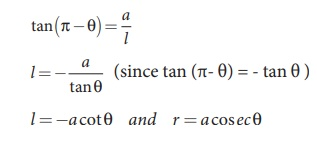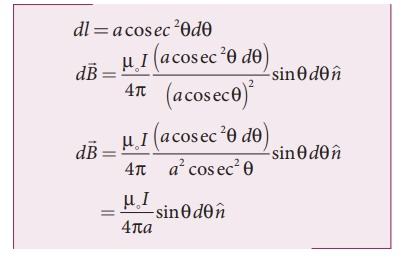Home | | Physics 12th Std | Magnetic field due to long straight conductor carrying current

# Magnetic field due to long straight conductor carrying current

Biot - Savart Law: Magnetic field due to long straight conductor carrying current

Magnetic field due to long straight conductor carrying currentConsider a long straight wire NM with current I flowing from N to M as shown in Figure 3.39. Let P be the point at a distance a from point O. Consider an element of length dl of the wire at a distance l from point O andbe the vector joining the element dl with the point P. Let θ be the angle betweenand. Then, the magnetic field at P due to the element isThe direction of the field is perpendicular to the plane of the paper and going into it. This can be determined by taking the cross product between two vectorsand(let it be n^ ). The net magnetic field can be determined by integrating equation (3.38) with proper limits.

From the Figure 3.39, in a right angle triangle PAO,Differentiating,This is the magnetic field at a point P due to the current in small elemental length. Note that we have expressed the magnetic field OP in terms of angular coordinate i.e. θ. Therefore, the net magnetic field at the point P which can be obtained by integrating dby varying the angle from θ = φ1 to θ = φ2 isFor a an infinitely long straight wire, ϕ1 = 0 and ϕ2 = π, the magnetic field isNote that here n ^ represents the unit vector from the point O to P.

EXAMPLE 3.15

Calculate the magnetic field at a point P which is perpendicular bisector to current carrying straight wire as shown in figure.Solution

Let the length MN = y and the point P is on its perpendicular bisector. Let O be the point on the conductor as shown in figure.The result obtained is same as we obtained in equation (3.39).

EXAMPLE 3.16

Show that for a straight conductor, the magnetic fieldSolution:

In a right angle triangle OPN, let the angle  OPN = θ1 which implies, ϕ1 = π /2  − θ1 and also in a right angle triangle OPM, OPM = θ2 which implies, ϕ2 = π/2 + θ2

Hence,Tags : Biot - Savart Law | Physics , 12th Physics : Magnetism and Magnetic Effects of Electric Current
Study Material, Lecturing Notes, Assignment, Reference, Wiki description explanation, brief detail
12th Physics : Magnetism and Magnetic Effects of Electric Current : Magnetic field due to long straight conductor carrying current | Biot - Savart Law | Physics